# Introduction to waves#

Waves

In this notebook, wave properties will be discussed, as well as derivations for simple harmonic motion and the wave equation.

import numpy as np
import matplotlib.pyplot as plt


## Wave properties#

A summary of the wave properties can be seen in the figure below: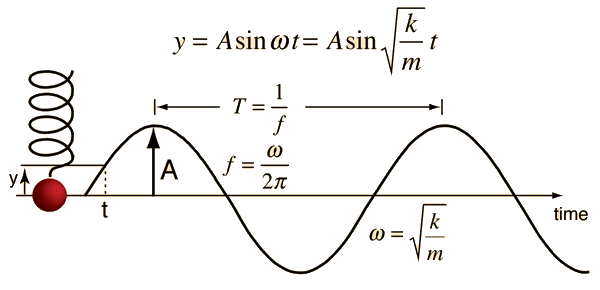Selection of wave properties:

• $$t$$ – time

• $$A$$ – amplitude

• $$f$$ – frequency, $$f = \frac{\omega}{2\pi}$$

• $$\lambda$$ – wavelength, $$\lambda = vf = \frac{2\pi}{k}$$

• $$T$$ – period, $$T = \frac{1}{f}$$

• $$p$$ – phase shift

• $$\omega$$ – angular frequency

• $$k$$ – wave number

• $$c_p$$ – phase speed, $$c_p = \frac{\omega}{k}$$

A variation and comparison between a selection of wave properties can be seen below:

def sine_wave(t, A, f, p):
"""
This formula will be derived later on for simple harmonic motion using Newton's 2nd law
"""
y = A*np.sin(2*np.pi*f*t + p*np.pi/180)
return y

t = np.linspace(0,5,200)

A1 = 2
f1 = 1
p1 = 1
y1 = sine_wave(t, A1, f1, p1)

A2 = 1.5
f2 = 2
p2 = 0.2
y2 = sine_wave(t, A2, f2, p2)

plt.figure(figsize=(12,5))
plt.xlabel("Time (s)")
plt.plot(t, y1)
plt.plot(t, y2)
plt.grid(True)
plt.show()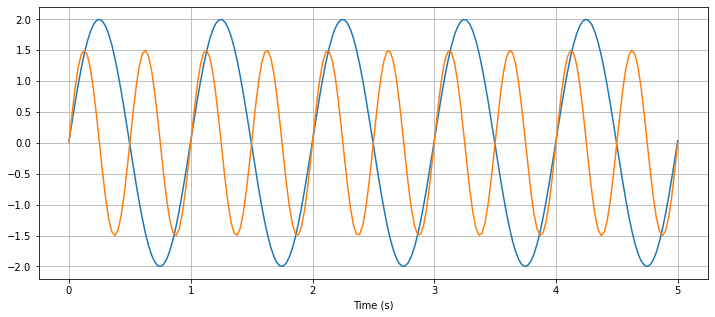## Simple Harmonic Motion#

A wave carries information and energy but does not transport the material. It undergoes simple harmonic motion.

To derive the formula for simple harmonic motion (SHM), assume an object of mass m and mean position $$y_0$$ and the displacement ‘$$y$$’. In SHM, there is a restoring force that is directed towards the mean position $$y_0$$ at every instant. The restoring force is directly proportional to the displacement of the body at every instant, so $$F \propto -y$$. The negative sign indicates that the restoring force acts opposite to the displacement. Thus, including the force constant, $$k$$,

$F = -ky \qquad (1)$

Now consider Newton’s second law of motion $$F = ma$$, where $$F$$ is the restoring force on the body, $$m$$ is the mass and $$a$$ is the acceleration.

Since $$a = \frac{dv}{dt}= \frac{d^2 y}{d t^2}$$, so

$F = m \frac{d^2 y}{d t^2} \qquad (2)$

Combining equations (1) and (2), we get

$m \frac{d^2 y}{d t^2} = -ky$
$\frac{d^2 y}{d t^2} = - \frac{k}{m}y$
$\frac{d^2 y}{d t^2} + \frac{k}{m}y = 0$

Let $$\frac{k}{m} = \omega^2$$. Now,

$\frac{d^2 y}{d t^2} + \omega^2 y = 0 \qquad (3)$

The solution to this equation can be approximated from analysis, knowing that a sin or cosine function when differentiated twice returns to it’s original form, with a factor in front. So, we can estimate the solution to be

$y = A \sin(\omega t)$

As we know that

$v = \frac{dy}{dt} = A\omega \sin(\omega t)$

and

$a = \frac{d^2y}{dt^2} = A\omega^2 \sin(\omega t)$

Filling $$y = A \sin(\omega t)$$ back into equation (3), we get

$A\omega^2 \sin(\omega t) + A \sin(\omega t) \cdot \omega^2$

which shows that this solution is valid.

Thus, the solution to the wave equation is:

$y = A \sin(\omega t) = A \sin (2 \pi f t)$

Here, $$A$$ is the magnitude of the initial displacement, $$\omega$$ is the angular frequency and $$t$$ is time.

def displacement(t, A, f):
y = A*np.sin(2*np.pi*f*t)
return y

def velocity(t, A, f):
v = A*2*np.pi*f*np.cos(2*np.pi*f*t)
return v

def acceleration(t, A, f):
a = A*(2*np.pi*f)**2*np.sin(2*np.pi*f*t)
return a

t = np.linspace(0,5,200)

A = 2
f = 1
displacement = displacement(t, A, f)
velocity = velocity(t, A, f)
acceleration = acceleration(t, A, f)

plt.figure(figsize=(12,5))
plt.xlabel("Time (s)")
plt.plot(t, displacement, label = "displacement (m)")
plt.plot(t, velocity, label = "velocity (m/s)")
plt.plot(t, acceleration, label = "acceleration ($m^2$/s)")
plt.grid(True)
plt.legend()
plt.title("Relationship of displacement, velocity and acceleration")
plt.show()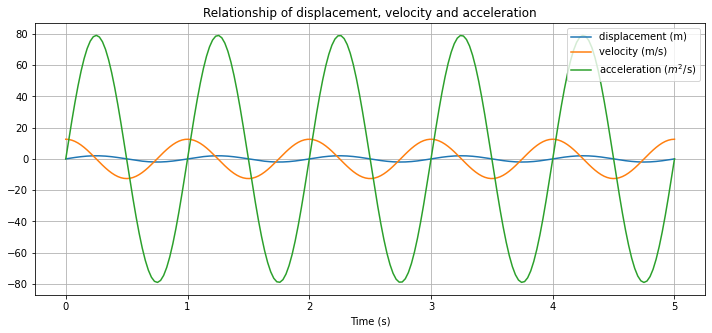Note a few things in the plot above:

• Velocity is zero where particle displacement is at it’s maximum or minimum.

• Velocity is at it’s maximum or minimum when the particle displacement goes through the equilibrium position.

• When particle displacement is at the equilibrium position, acceleration is zero.

• Acceleration has a maximum or minimum when the particle displacement has a maximum or minimum.

Using trigonometry,

$F = ma = -mg\sin(\theta)$

Thus,

$a = g\sin(\theta)$

Approximate that for small $$\sin(\theta) = \theta$$, so

$F = ma = mg\theta = m \frac{y}{l}$

Thus,

\begin{align}\begin{aligned}a = \frac{F}{ml}y (tussenstap). \\If the restoring force is tension from a string for example, it can be approximated as F=-2T, considering a string pulls the body from the left and right side.\\The equation of simple harmonic motion then becomes: \\\frac{d^2 y}{d t^2} = - \frac{2T}{ml} y = -\omega ^2 y \end{aligned}\end{align}

## Wave equation#

To derive the wave equation, a string with density $$\rho$$ per unit length is stretched in the $$x$$ direction with tension force $$T$$. A wave can be described as coupled simple harmonic motion. This can be thought of as a rope with lots of masses being displaced individually.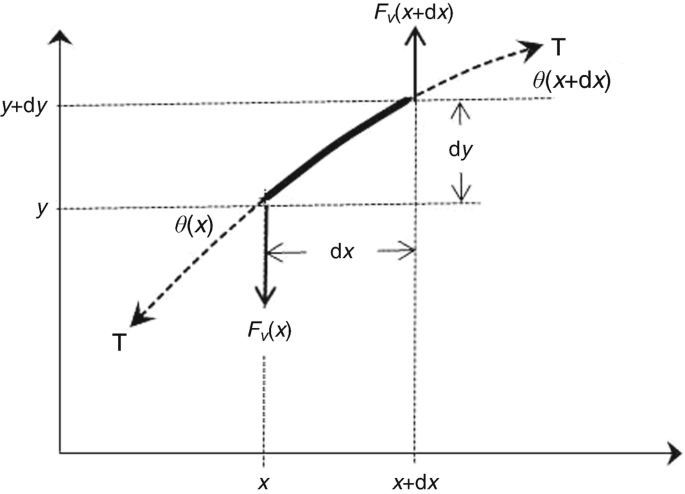The vertical forces on the string must balance.

Consider Newton’s second law for the force, where $$F=ma$$

Consider $$m=\rho\Delta x$$, as $$m = \rho V$$ and only one dimension is considered. So, force is

$ma=\rho \Delta x \cdot \frac{\partial ^2 u}{\partial t^2}$

This is equal to the difference between the vertical tension T at the two ends, so

$\rho \Delta x \cdot \frac{\partial ^2 u}{\partial t^2} = T(x+ \Delta x, t)\sin\left[\theta(x+\Delta x, t)\right] - T(x,t)\sin\left[\theta(x,t)\right]$

Assume that the deflection angles $$\theta$$ are small, $$\sin\theta = \tan\theta = \frac{\partial u}{\partial x}$$. Thus,

$\rho \Delta x \cdot \frac{\partial ^2 u}{\partial t^2} = T(x+ \Delta x, t)\frac{\partial u}{\partial x} - T(x,t)\frac{\partial u}{\partial x}$

Divide both sides by $$\Delta x$$ and letting $$\Delta x \rightarrow 0$$ gives:

$\rho(x)\frac{\partial^2 u}{\partial t^2} = \frac{\partial}{\partial x}\left(T \frac{\partial u}{\partial x}\right)$

Assuming deflection is small, $$T$$ can be assumed to be constant.

Considering $$c^2 = \frac{T}{\rho}$$, the wave equation becomes

$\frac{\partial^2 u}{\partial t^2} = c^2 \frac{\partial^2 u}{\partial x^2}$

One solution to the wave equation is by using travelling waves of the form $$u(x,t) = f(x \pm ct)$$.

At $$t=0$$, $$f(x)$$ is a shape in space.

$$f(x-ct)$$ is the shape of $$f(x)$$ when travelling to the right at speed $$c$$.

$$f(x+ct)$$ is the shape of $$f(x)$$ when travelling to the left at speed $$c$$.To plot the wave equation, a few initial conditions have to be set. consider

## Types of waves#

Body waves

• Longitudinal waves: waves where the displacement of the medium is in the same direction as the travelling wave. Examples are P-waves or sound waves.

• Transverse wave: waves where motion of all points oscillate in a path that is perpendicular to the direction of the travelling wave. Examples are seismic S-waves, electro-magnetic waves and vibrations on string.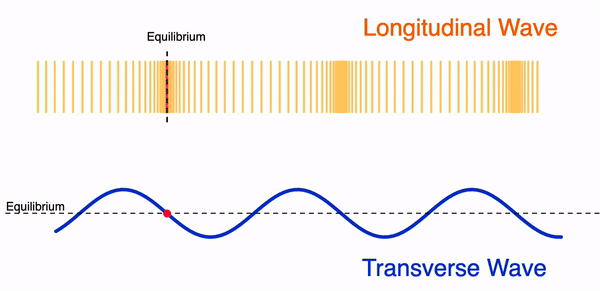Surface waves

• Love wave: wave moving horizontally and perpendicular to the direction of travel.• Rayleigh wave: wave causing elliptical retrograde motion (opposite to direction of travel) in the vertical plane along direction of travel as a combination of both longitudinal and transverse vibration.• Water wave: circular motion of elements moving in the same direction of wave travel.